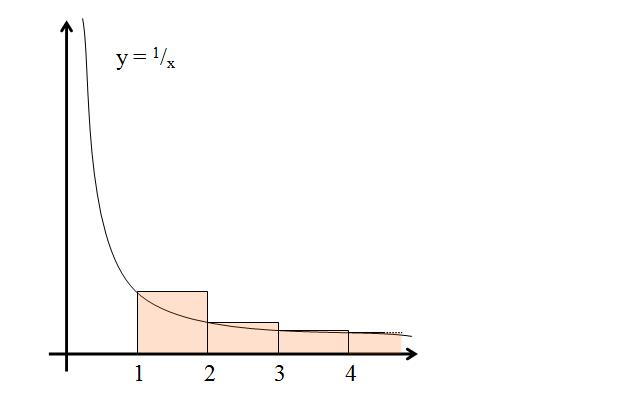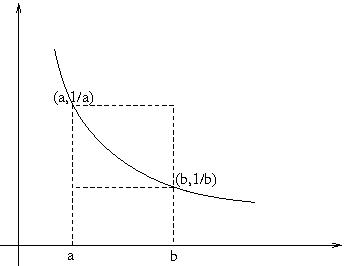#### You may also like### Telescoping Series

Find $S_r = 1^r + 2^r + 3^r + ... + n^r$ where r is any fixed positive integer in terms of $S_1, S_2, ... S_{r-1}$.### Degree Ceremony

What does Pythagoras' Theorem tell you about these angles: 90Â°, (45+x)Â° and (45-x)Â° in a triangle?### OK! Now Prove It

Make a conjecture about the sum of the squares of the odd positive integers. Can you prove it?

# Harmonically

##### Age 16 to 18Challenge Level

The Harmonic Series.

Murat Aygen from Turkey sent this solution to part (a) and Dapeng Wang from Claremont Fan Court School, Guildford sent in essentially the same solution.

Let us partition the terms of our series, starting from every $k= 2^{i-1}+ 1$, as follows: $\sum_{k=1}^{2^r} \left({1\over{k}}\right) = 1 + \left({1\over{2}}\right) + \left({1\over{3}} + {1\over{4}}\right) + \left({1\over{5}} + {1\over{6}} + {1\over{7}} + {1\over{8}}\right) + \sum_{i=4}^{r} \left(\frac{1}{2^{i-1}+1} + \dots + {1\over2^{i}}\right)$

How many terms are there in each partition? We see that there are 1, 2, 4, 8, 16... terms in the successive partitions given by $2^i - 2^{i -1}$ where $2^i - 2^{i -1} = 2^{i -1}(2-1) = 2^{i -1}$.

The smallest term in a partition is clearly the rightmost term ${1\over 2^i}$. This smallest term multiplied by the number of terms in the partition is equal to $${1\over 2^i}\times 2^{i -1} = {1\over 2}$$ which is always less than the sum of the terms of the partition. Anyway it is a positive constant! Since the number of terms in the series is as many as one wishes, we can form as many partitions as we wish whose partial sums are not less than 1/2. For reaching a sum of 100 only 200 partitions are needed.

Noah and Ariya from The British School of Boston, USA and Aled from King Edward VI Camp Hill School for Boys sent excellent solutions to part (b) .Each of the areas of the pink rectangles is representative of one fraction in the sum
$$S_n = 1 +{1\over 2} + {1\over 3} + {1\over 4} + ... + {1\over n}.$$This is verified by knowing that each rectangle x has a base of length 1 and a height of length 1/x. Every rectangle has an area greater than that under the curve $y=1/x$ it overlaps, as illustrated above (note that this will remain so because the function $y= 1/x$ is monotonic for positive numbers).Consider the area under the graph $y = 1/x$ between $x=a$ and $x=b$. This area lies between two rectangles and so we get $${b-a \over b} < \int_a^b{ 1\over x }dx = \ln b - \ln a < { b-a\over a}$$ If we evaluate the expression between $a = {1\over n}$ and $b= {1 \over {n-1}}$ we get:

$${1\over n} < \ln {1 \over {n-1}} - \ln {1 \over n} = \ln n - \ln (n-1) < { 1\over n-1}$$
and this gives:
$${1 \over 2} < \ln 2- \ln 1< { 1\over 1}$$
$${1 \over 3} < \ln 3- \ln 2< { 1\over 2}$$
$${1 \over 4} < \ln 4- \ln 3< { 1\over 3}$$
...and so on
$${1 \over n} < \ln n- \ln (n-1)< { 1\over n-1}$$
Summing these expressions (noting that $\ln1 = 0$) we get:
$${1\over 2} + {1\over 3} + {1\over 4} + ... + {1\over n} < \ln n < 1 + {1\over 2} + {1\over 3} + {1\over 4} + ... + {1\over n-1} .$$
The series on each side of this inequality grow infinitely large and differ by less than 1 so the series grows like $\ln n$.